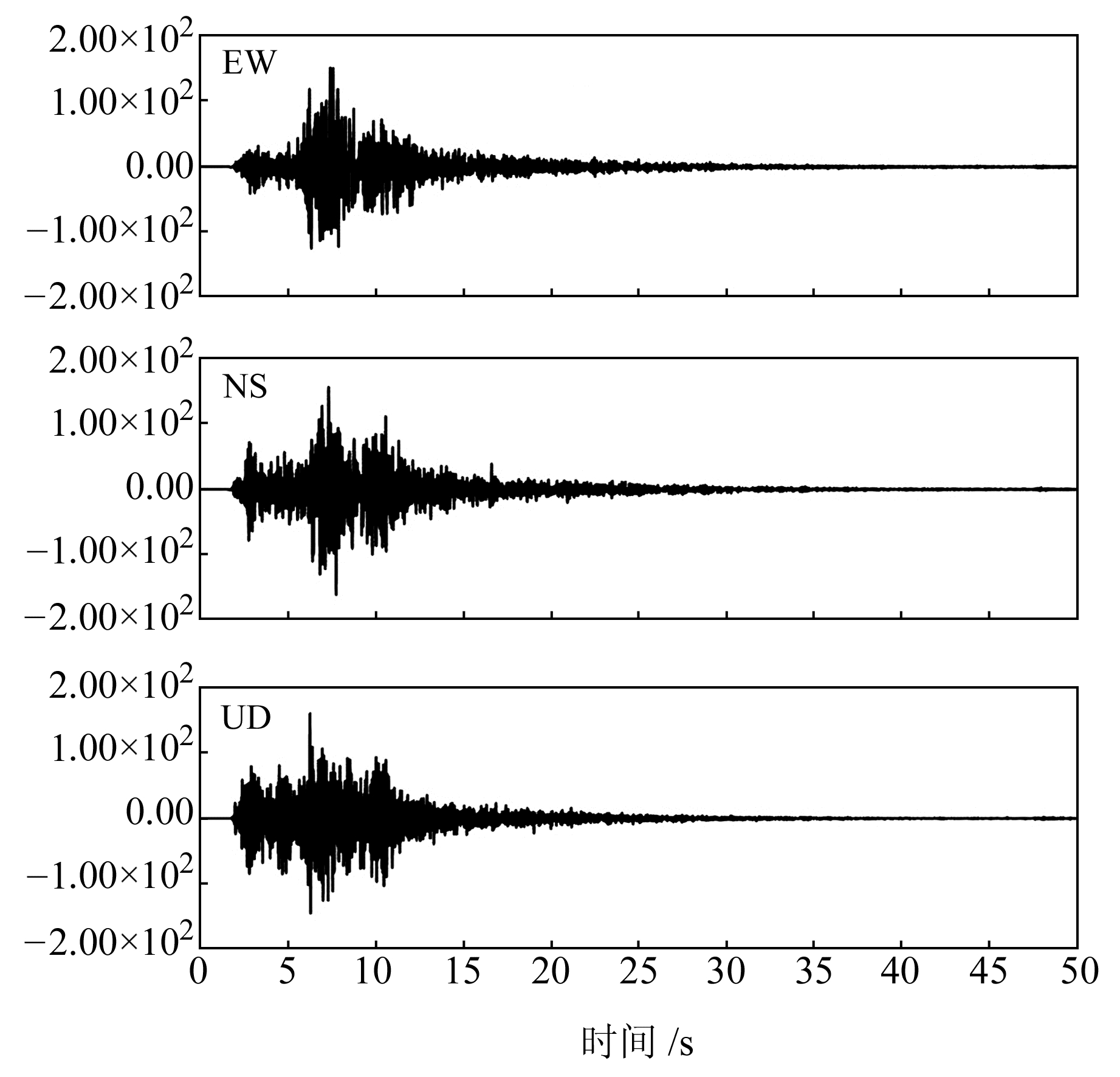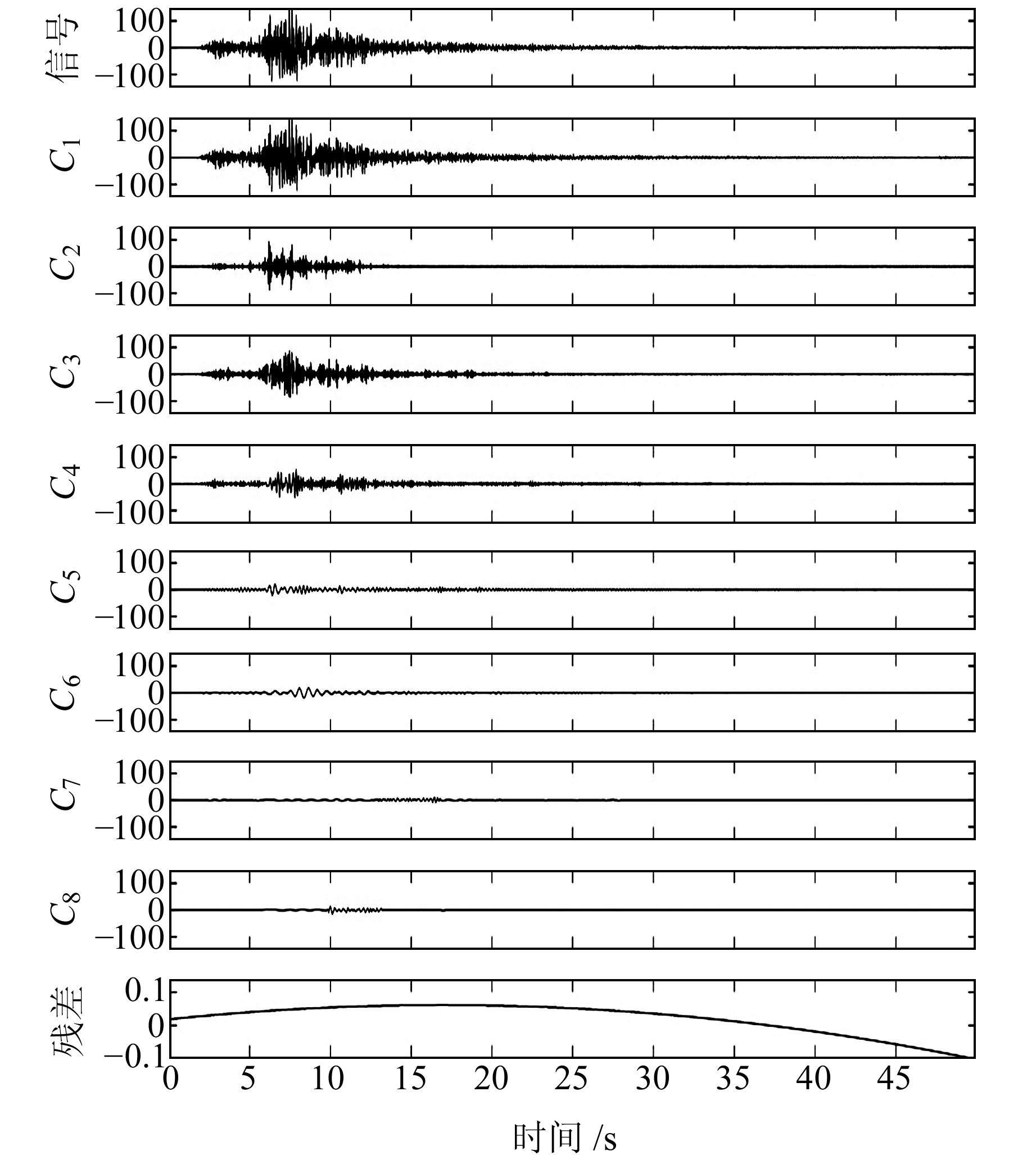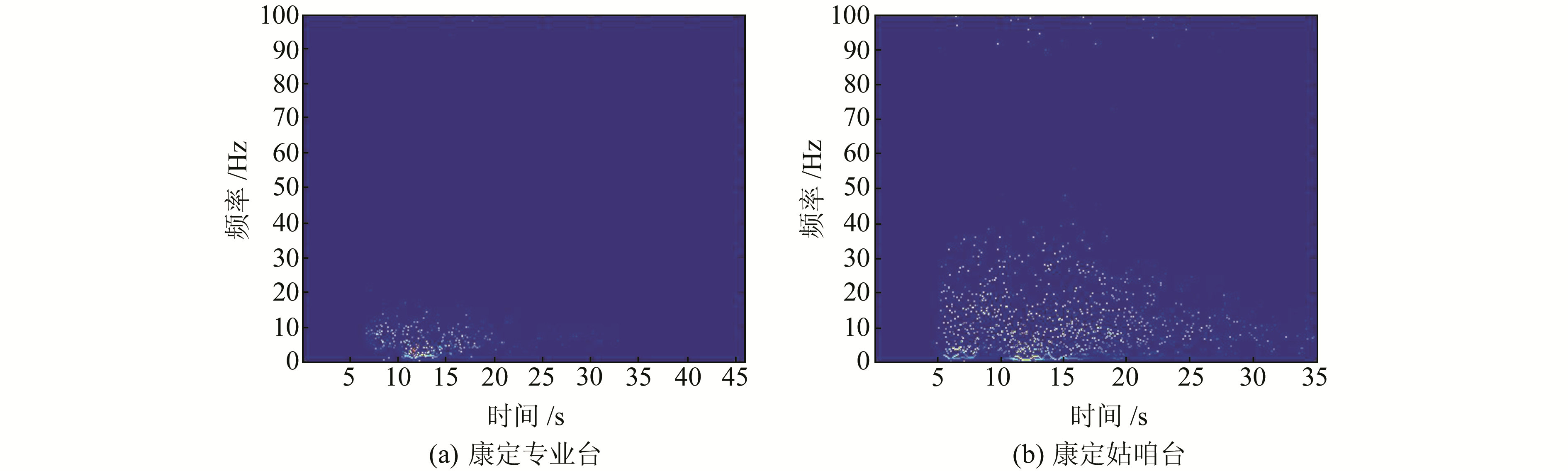﻿ 2014-11-22康定M<sub>S</sub>6.3地震加速度记录的时频分析与震害特征文章快速检索 高级检索
 大地测量与地球动力学2019, Vol. 39Issue (8): 875-880  DOI: 10.14075/j.jgg.2019.08.020### 引用本文LIANG Hong, LI Dahu, CHEN Xuefen, et al. Time-Frequency Analysis and Earthquake Damage Characteristics of Acceleration Records from Kangding MS6.3 Earthquake on Nov 22, 2014[J]. Journal of Geodesy and Geodynamics, 2019, 39(8): 875-880.### Foundation support

Science and Technology Innovation Team Fund of Sichuan Earthquake Agency, No.201804.

### 第一作者简介

LIANG Hong, engineer, majors in earthquake observation and strong earthquake data processing, E-mail:583108668@qq.com.

### 文章历史

2014-11-22康定MS6.3地震加速度记录的时频分析与震害特征

1. 四川省地震局，成都市人民南路三段29号，610041

2014-11-22四川省甘孜藏族自治州康定县发生MS6.3地震(30.3°N，101.7°E)，震源深度18 km，震中位于鲜水河断裂南段。根据四川台网地震目录，截至27日16时，共记录到余震1 544次，其中5.0~5.9级1次，2.0~2.9级6次，最大余震为2014-11-25发生的5.8级地震，余震优势分布方向为NW向。四川省地震局在全省范围内布设的数字强震监测台网记录到了此次地震，最大峰值加速度记录为距震中34.7 km的康定新都台，获取的SN向分量为161.65 cm/s2(台站分布见图 1)。提取和分析康定地震加速度记录的时频特性，对深入研究地震波的传播路径和过程机理具有重要意义[1-2]图 1 区域断裂分布和台站分布 Fig. 1 Regional fault and the distribution of stations

1 加速度记录的分析方法

Hilbert变换和谱分析为：$\hat c{_i}\left( t \right) = {c_i}\left( t \right)\frac{1}{{{\rm{ \mathsf{ π} }}t}} = $$\int_{ + \infty }^{ + \infty } {{c_i}\left( {t' } \right) \frac{1}{{\pi \left( {t - t' } \right)}}}{\rm{d}}\mathit{t}= \int_{ + \infty }^{ + \infty } {{c_i}\left( {t - t' } \right)\frac{{1}}{{{\rm{ \mathsf{ π} }}t' }}{\rm{d}}\mathit{t}} ，构造原信号的解析信号为：{z_i}\left( t \right) = {c_i}\left( t \right) + i{{\hat c}_i}\left( t \right) = {a_i}\left( t \right){{\rm{e}}^{{\rm{j}}{\theta _i}(t)}}, {a_i}\left( t \right) = \sqrt {{c_i}{{\left( t \right)}^2} + {{\hat c}_i}{{\left( t \right)}^2}}$${\theta _i}\left( t \right) = {\rm{arctan}}(\frac{{{{\hat c}_i}\left( t \right)}}{{{c_i}\left( t \right)}})$，则瞬时频率为${\omega _i}\left( t \right) = \frac{{{\rm{d}}{\theta _i}\left( t \right)}}{{{\rm{d}}\mathit{t}}}$。于是可以得到${z_i}\left( t \right) = {c_i}\left( t \right) + i{{\hat c}_i}\left( t \right) = {a_i}\left( t \right){{\rm{e}}^{{\rm{j}}{\theta _i}(t)}} = {a_i}\left( t \right){{\rm{e}}^{{\rm{j}}\int_0^T {{\omega _i}\left( t \right){\rm{d}}\mathit{t}} }}$，进而得到$x\left( t \right) = \sum\limits_{i = 1}^n {{c_i}\left( t \right)} = {\rm{Re}}\sum\limits_{i = 1}^n {{z_i}\left( t \right)}$$= {\rm{Re}}\sum\limits_{i = 1}^n {{a_i}\left( t \right){{\rm{e}}^{{\rm{j}}{\theta _i}(t)}}} = {\rm{Re}}\sum\limits_{i = 1}^n {{a_i}\left( t \right){{\rm{e}}^{{\rm{j}}\int_0^T {{\omega _i}\left( t \right){\rm{d}}\mathit{t}} }}}$。因此，Hilbert谱为$H\left( {\omega , t} \right) = {\rm{Re}}\sum\limits_{i = 1}^n {{a_i}\left( t \right){{\rm{e}}^{{\rm{j}}\int_0^T {{\omega _i}\left( t \right){\rm{d}}\mathit{t}} }}}$。再定义Hilbert边际谱为$h\left( \omega \right) = \int_{ - \infty }^{ + \infty } {H\left( {\omega , t} \right){\rm{d}}\mathit{t}}$，瞬时能量谱为${\rm{IE}}\left( t \right) = \int_\infty {{H^2}\left( {\omega , t} \right){\rm{d}}} \omega$，则Hilbert能量谱为${\rm{ES}}\left( \omega \right) = \int_0^T {{H^2}\left( {\omega , t} \right){\rm{d}}\mathit{t}}$

2 加速度记录的时频分析 2.1 EEMD分解结果图 2 新都台加速度记录 Fig. 2 Acceleration records of Xindu station图 3 经过EEMD后的各IMF分量 Fig. 3 Each IMF component after EEMD表 1 各个IMF量化统计值 Tab. 1 The statistic of each IMF component
2.2 Hilbert变换和时频谱图 4 FFT谱和Hilbert边际谱 Fig. 4 Fast Fourier transformation spectrum and Hilbert marginal spectrum图 5 不同震中距台站记录的时频谱 Fig. 5 Time-frequency spectrum recorded by stations with different epicentral distances图 6 瞬时能量谱 Fig. 6 Instantaneous energy spectrum图 7 能量谱 Fig. 7 The energy spectrum
3 震害特征分析图 8 康定MS6.3地震典型震害照片 Fig. 8 Typical earthquake damage photographs of Kangding MS6.3 earthquake
4 结语

1) 本文选取康定MS6.3地震中不同震中距台站记录的三分量加速度数据，并采用基于EEMD的HHT方法对其进行时频分析。结果表明，EEMD不仅能抑制信号的模态混叠，还能较好地计算数据的时频特性，对能量集中分布的时频段也能较好地体现。

2) 根据地震现场工作科学考察发现，震区崩科藏式建筑房屋表现出震害明显偏重的现象，其原因可能与几个因素有关：首先，通过Hilbert边际谱分析可以看出，康定新都台信号频率集中在10 Hz左右，瞬时能量谱显示高频成分丰富；其次，震害分布特征可能还与房屋建筑结构有关，农牧区的崩科式建筑房屋抗震能力差，损毁较严重，城镇区框架结构房屋损毁程度较轻。目前针对崩科藏式结构房屋抗震设计的研究较少，今后需采用量化分析的方式，从结构动力学角度对房屋结构的刚度、阻尼等基本参数进行研究，记录其在遭遇破坏性地震后相关参数的变化情况和特征，为此类型房屋的抗震设计工作提供可靠的科学依据。

  李小军, 周正华, 于海英, 等.汶川8.0级地震强震动观测及记录初步分析[C].汶川地震建筑震害分析与重建研讨会, 北京, 2008 (Li Xiaojun, Zhou Zhenghua, Yu Haiying, et al. Preliminary Analysis of Strong Ground Motion Observation for Wenchuan MS8.0 Earthquake[C]. Seminar on Building Damage Analysis and Reconstruction in Wenchuan Earthquake, Beijing, 2008) http://cpfd.cnki.com.cn/Article/CPFDTOTAL-OGTY200806001004.htm (0)  李大虎, 赖敏, 何强, 等. 基于聚类经验模态分解(EEMD)的汶川MS8.0强震动记录时频特性分析[J]. 地震学报, 2012, 34(3): 350-362 (Li Dahu, Lai Min, He Qiang, et al. Time-Frequency Characteristics Analysis of Wenchuan MS8.0 Strong Motion Records Based on EEMD Decomposition[J]. Acta Seismologica Sinica, 2012, 34(3): 350-362 DOI:10.3969/j.issn.0253-3782.2012.03.007) (0)  Daubechies I. The Wavelet Transform, Time-Frequency Localization and Signal Analysis[J]. IEEE Transactions on Information Theory, 1990, 36(5): 961-1 005 DOI:10.1109/18.57199 (0)  李大虎, 梁明剑, 黎小刚, 等. 011年四川炉霍MS5.3地震加速度记录的时频分析与能量计算[J]. 西北地震学报, 2012, 34(4): 335-341 (Li Dahu, Liang Mingjian, Li Xiaogang, et al. Time-Frequency Analysis and Energy Calculation for Acceleration Records of Luhuo MS5.3 Earthquake in Sichuan Province in 2011[J]. Northwestern Seismological Journal, 2012, 34(4): 335-341) (0)  郝国成, 龚婷, 董浩斌, 等. 基于聚类经验模态分解的地球天然脉冲电磁场时频与能量谱分析:以芦山MS7.0地震为例[J]. 地学前缘, 2015, 22(5): 231-238 (Hao Guocheng, Gong Ting, Dong Haobin, et al. Time-Frequency Characteristics and Energy Analysis of the Earth's Natural Pulse Electromagnetic Fields Based on Ensemble Empirical Mode Decomposition: The Lushan MS7.0 Earthquake as an Example[J]. Earth Science Frontiers, 2015, 22(5): 231-238) (0)  Krishna B T. Electrocardiogram Signal and Linear Time-Frequency Transforms[J]. Journal of the Institution of Engineers(India): Series B, 2014, 95(4): 377-382 DOI:10.1007/s40031-014-0097-9 (0)  Zhou Y H, Chen W C, Gao J H, et al. Application of Hilbert-Huang Transform Based Instantaneous Frequency to Seismic Reflection Data[J]. Journal of Applied Geophysics, 2012, 82: 68-74 DOI:10.1016/j.jappgeo.2012.04.002 (0)  Wu Z H, Huang N E. Ensemble Empirical Mode Decomposition:A Noise-Assisted Data Analysis Method[J]. Advances in Adaptive Data Analysis, 2009, 1(1): 1-41 (0)  Wu Z H, Huang N E. A Study of the Characteristics of White Noise Using the Empirical Mode Decomposition Method[J]. Proceedings of the Royal Society of London Series A: Mathematical Physical and Engineering Sciences, 2004, 460(2 046): 1 597-1 611 (0)  Huang N E, Shen Z, Long S R, et al. The Empirical Mode Decomposition and the Hilbert Spectrum for Nonlinear and Non-Stationary Time Series Analysis[J]. Proceedings of the Royal Society of London Series A: Mathematical Physical and Engineering Sciences, 1998, 454(1 971): 903-995 (0)  Lu Z, Smith J S, Wu Q H, et al. Empirical Mode Decomposition for Power Quality Monitoring[C]. 2005 IEEE/PES Transmission and Distribution Conference and Exposition: Asia and Pacific, Dalian, 2005 http://ieeexplore.ieee.org/document/1546916/ (0)  李大虎, 李军, 邓艳, 等. 云南香格里拉、德钦-四川得荣交界5.9级地震加速度记录的时频分析[J]. 物探化探计算技术, 2015, 37(6): 716-723 (Li Dahu, Li Jun, Deng Yan, et al. Time-Frequency Analysis of MS5.9 Earthquake Acceleration Records in Border of Xianggelila between Deqin of Yunnan and Derong of Sichuan[J]. Computing Techniques for Geophysical and Geochemical Exploration, 2015, 37(6): 716-723 DOI:10.3969/j.issn.1001-1749.2015.06.08) (0)  易桂喜, 龙锋, 闻学泽, 等. 2014年11月22日康定M6.3级地震序列发震构造分析[J]. 地球物理学报, 2015, 58(4): 1 205-1 219 (Yi Guixi, Long Feng, Wen Xueze, et al. Seismogenic Structure of the M6.3 Kangding Earthquake Sequence on 22 Nov. 2014, Southwestern China[J]. Chinese Journal of Geophysics, 2015, 58(4): 1 205-1 219) (0)  周荣军, 赖敏, 李大虎, 等. 2011年4月10日四川炉霍Ms5.3级地震强震记录与震害特点[J]. 成都理工大学学报:自然科学版, 2013, 40(2): 217-224 (Zhou Rongjun, Lai Min, Li Dahu, et al. Strong-Motion Records and Characteristics of Earthquake Damages of MS5.3 Earthquake Occurred in Luhuo, Sichuan, China, on April 10, 2011[J]. Journal of Chengdu University of Technology: Science and Technology Edition, 2013, 40(2): 217-224) (0)  2014年11月22日四川省甘孜藏族自治州康定县6.3级地震灾害直接损失评估报告[R].四川省地震局, 2011 (Assessment Report on Direct Damage of Earthquake Disaster with MS6.3 in Kangding County, Ganzi Tibetan Autonomous Prefecture, Sichuan Province, 22 November 2014[R].Sichuan Earthquake Agency, 2011) (0)
Time-Frequency Analysis and Earthquake Damage Characteristics of Acceleration Records from Kangding MS6.3 Earthquake on Nov 22, 2014
LIANG Hong1LI Dahu1     CHEN Xuefen1     LIU Xuemei1     CHEN Bing1     ZHAO Jing1     ZHAO Tianxia1
1. Sichuan Earthquake Agency, 29 Section 3, South-Renmin Road, Chengdu 610041, China
Abstract: The HHT method based on clustering empirical mode decomposition(EEMD) is used to extract the time-frequency characteristics of the strong earthquake record of Kangding MS6.3 earthquake. The EEMD and Hilbert transform and spectrum analysis are performed on the strong vibration acceleration records acquired by each station. We compare with the Fourier transform. The results show that for nonlinear strong earthquake recording, EEMD can effectively suppress the modal aliasing problem existing in EMD. The results of the earthquake damage investigation in Kangding earthquake area show that the seismic damage characteristics of the collapsed building houses are more serious. It can be seen from the Hilbert marginal spectrum analysis that the main frequency of the stations in the earthquake zone is concentrated at around 10 Hz, and the instantaneous energy spectrum is high. The information is richer, and the poor seismic resistance of Tibetan buildings is also an important reason for the earthquake-damaged Tibetan-style structural houses.
Key words: EEMD; HHT; acceleration records; time-frequency characteristics; Hilbert spectrum; earthquake damage characteristics